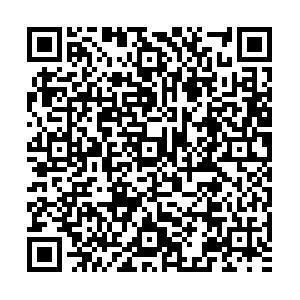# QCD analysis of CMS W + charm measurements at LHC with ${\sqrt { s} = 7\; {\bf{TeV}}}$and implications for strange PDF• We calculate cross-sections and cross-section ratios of a charm quark production in association with a W gauge boson at next-to-leading order QCD using MadGraph and CT10NNLO, CT14NNLO, and MSTW2008NNLO PDFs. We compare the results with measurements from the CMS detector at the LHC at a center-of-mass energy of 7 TeV. Moreover, we calculate absolute and normalized differential cross-sections as well as differential cross-section ratios as a function of the lepton pseudorapidity from the W boson decay. The correlation between the CT14NNLO PDFs and predictions for $W+$charm data are studied as well. Furthermore, by employing the error PDF updating method proposed by the CTEQ-TEA group, we update CT14NNLO PDFs, and analyze the impact of CMS 7 TeV $W+$charm production data to the original CT14NNLO PDFs. By comparison of the $g(x,Q)$, $s(x,Q)$, $u(x,Q)$, $d(x,Q)$, $\bar u(x,Q)$, and $\bar d(x,Q)$PDFs at $Q=1.3$GeV and $Q = 100$GeV for the CT14NNLO and CT14NNLO+Wc, we see that the error band of the $s(x,Q)$PDF is reduced in the region $x<0.4$, and the error band of $g(x,Q)$PDF is also slightly reduced at region $0.01 < x<0.1$.
••Get Citation
Nijat Yalkun and Sayipjamal Dulat. QCD analysis of CMS W + charm measurements at LHC with ${\sqrt { s} = 7\; {\bf{TeV}}}$and implications for strange PDF[J]. Chinese Physics C, 2019, 43(12): 123101. doi: 10.1088/1674-1137/43/12/123101
Nijat Yalkun and Sayipjamal Dulat. QCD analysis of CMS W + charm measurements at LHC with ${\sqrt { s} = 7\; {\bf{TeV}}}$and implications for strange PDF[J]. Chinese Physics C, 2019, 43(12): 123101.Milestone
Revised: 2019-10-09
Article Metric

Article Views(442)
Cited by(0)
Policy on re-use
To reuse of Open Access content published by CPC, for content published under the terms of the Creative Commons Attribution 3.0 license (“CC CY”), the users don’t need to request permission to copy, distribute and display the final published version of the article and to create derivative works, subject to appropriate attribution.
###### 通讯作者: 陈斌, bchen63@163.com
• 1.

沈阳化工大学材料科学与工程学院 沈阳 110142

Title:
Email:

## QCD analysis of CMS W + charm measurements at LHC with ${\sqrt { s} = 7\; {\bf{TeV}}}$and implications for strange PDF

###### Corresponding author: Sayipjamal Dulat, sdulat@hotmail.com
• 1. School of Physics Science and Technology, Xinjiang University, Urumqi, Xinjiang 830046 China
• 2. Institute of Theoretical Physics, Chinese Academy of Sciences, Beijing 100190, China

Abstract: We calculate cross-sections and cross-section ratios of a charm quark production in association with a W gauge boson at next-to-leading order QCD using MadGraph and CT10NNLO, CT14NNLO, and MSTW2008NNLO PDFs. We compare the results with measurements from the CMS detector at the LHC at a center-of-mass energy of 7 TeV. Moreover, we calculate absolute and normalized differential cross-sections as well as differential cross-section ratios as a function of the lepton pseudorapidity from the W boson decay. The correlation between the CT14NNLO PDFs and predictions for $W+$charm data are studied as well. Furthermore, by employing the error PDF updating method proposed by the CTEQ-TEA group, we update CT14NNLO PDFs, and analyze the impact of CMS 7 TeV $W+$charm production data to the original CT14NNLO PDFs. By comparison of the $g(x,Q)$, $s(x,Q)$, $u(x,Q)$, $d(x,Q)$, $\bar u(x,Q)$, and $\bar d(x,Q)$PDFs at $Q=1.3$GeV and $Q = 100$GeV for the CT14NNLO and CT14NNLO+Wc, we see that the error band of the $s(x,Q)$PDF is reduced in the region $x<0.4$, and the error band of $g(x,Q)$PDF is also slightly reduced at region $0.01 < x<0.1$.

### HTML关注分享DownLoad:  Full-Size Img  PowerPoint